# JP4556873B2 - Image collation system and image collation method - Google Patents

## Info

Publication number
JP4556873B2
JP4556873B2 JP2005514861A JP2005514861A JP4556873B2 JP 4556873 B2 JP4556873 B2 JP 4556873B2 JP 2005514861 A JP2005514861 A JP 2005514861A JP 2005514861 A JP2005514861 A JP 2005514861A JP 4556873 B2 JP4556873 B2 JP 4556873B2
Authority
JP
Japan
Prior art keywords
image
dimensional
reference image
reference
collation
Prior art date
Legal status (The legal status is an assumption and is not a legal conclusion. Google has not performed a legal analysis and makes no representation as to the accuracy of the status listed.)
Active
Application number
JP2005514861A
Other languages
Japanese (ja)
Other versions
JPWO2005038716A1 (en
Inventor

Original Assignee

Priority date (The priority date is an assumption and is not a legal conclusion. Google has not performed a legal analysis and makes no representation as to the accuracy of the date listed.)
Filing date
Publication date
Priority to JP2003360713 priority Critical
Priority to JP2003360713 priority
Application filed by 日本電気株式会社 filed Critical 日本電気株式会社
Priority to PCT/JP2004/015612 priority patent/WO2005038716A1/en
Publication of JPWO2005038716A1 publication Critical patent/JPWO2005038716A1/en
Application granted granted Critical
Publication of JP4556873B2 publication Critical patent/JP4556873B2/en
Application status is Active legal-status Critical
Anticipated expiration legal-status Critical

## Images

•••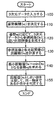•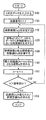•••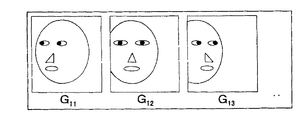•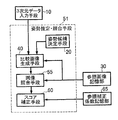•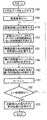•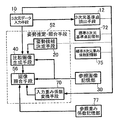•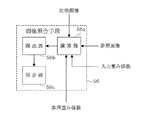•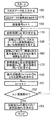•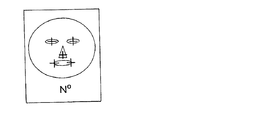•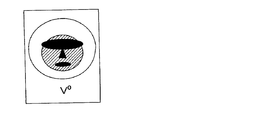•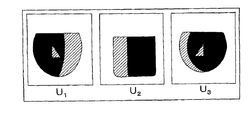•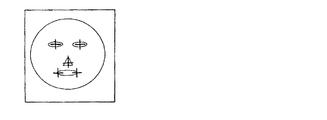•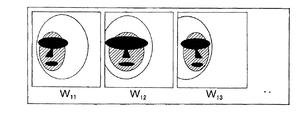•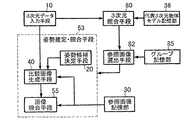•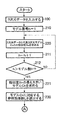•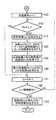•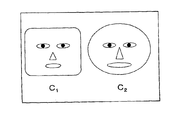•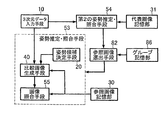•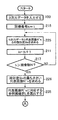•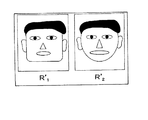••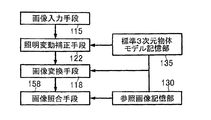•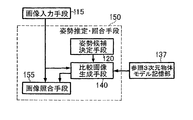## Classifications

• GPHYSICS
• G06COMPUTING; CALCULATING; COUNTING
• G06TIMAGE DATA PROCESSING OR GENERATION, IN GENERAL
• G06T15/003D [Three Dimensional] image rendering
• G06T15/10Geometric effects
• G06T15/20Perspective computation
• GPHYSICS
• G06COMPUTING; CALCULATING; COUNTING
• G06KRECOGNITION OF DATA; PRESENTATION OF DATA; RECORD CARRIERS; HANDLING RECORD CARRIERS
• G06K9/00Methods or arrangements for reading or recognising printed or written characters or for recognising patterns, e.g. fingerprints
• G06K9/00221Acquiring or recognising human faces, facial parts, facial sketches, facial expressions
• G06K9/00228Detection; Localisation; Normalisation
• G06K9/00248Detection; Localisation; Normalisation using facial parts and geometric relationships
• GPHYSICS
• G06COMPUTING; CALCULATING; COUNTING
• G06TIMAGE DATA PROCESSING OR GENERATION, IN GENERAL
• G06T7/00Image analysis
• G06T7/70Determining position or orientation of objects or cameras
• G06T7/73Determining position or orientation of objects or cameras using feature-based methods
• G06T7/74Determining position or orientation of objects or cameras using feature-based methods involving reference images or patches
• GPHYSICS
• G06COMPUTING; CALCULATING; COUNTING
• G06TIMAGE DATA PROCESSING OR GENERATION, IN GENERAL
• G06T2207/00Indexing scheme for image analysis or image enhancement
• G06T2207/30Subject of image; Context of image processing
• G06T2207/30196Human being; Person
• G06T2207/30201Face
• GPHYSICS
• G06COMPUTING; CALCULATING; COUNTING
• G06TIMAGE DATA PROCESSING OR GENERATION, IN GENERAL
• G06T2207/00Indexing scheme for image analysis or image enhancement
• G06T2207/30Subject of image; Context of image processing
• G06T2207/30232Surveillance

## Description

The present invention relates to an image collation system, an image collation method, and an image collation program. In particular, a three-dimensional model of an object cannot be registered in advance, and a reference image of each object in a database or network in the system is one. The present invention relates to an image collation system, an image collation method, and an image collation program that can collate and search with high accuracy even when there are only a few or a small number of images and images are taken under different conditions such as posture and illumination.

FIG. 25 is a block diagram showing a conventional image matching system. This conventional image collation system includes an image input unit 115, an image conversion unit 117, an image collation unit 157, a reference image storage unit 130, and a standard three-dimensional object model storage unit 135.

The reference image storage unit 130 stores in advance a reference image obtained by photographing an object. The standard three-dimensional object model storage unit 135 stores a standard three-dimensional object model in advance. The image conversion unit 117 converts the three-dimensional object model obtained from the standard three-dimensional object model storage unit 135 with respect to the common partial region of the input image input from the image input unit 115 and each reference image obtained from the reference image storage unit 130. The partial image is generated by converting the input image and / or the reference image so that the posture condition is the same.

The partial region is a characteristic part such as an eye, nose, or mouth, for example, and can be dealt with by designating feature points in advance for each image and the three-dimensional object model. The image matching unit 157 compares the input image converted by the image conversion unit 117 with the partial images of each reference image, calculates the average similarity, and selects the reference image having the highest similarity for each object (for example, JP 2000-322577 A (see Patent Document 1).

FIG. 26 is a block diagram showing another conventional image matching system. This conventional image collation system includes an image input unit 115, an illumination variation correction unit 122, an image conversion unit 118, an image collation unit 158, a reference image storage unit 130, and a standard three-dimensional object model storage unit 135. It is configured.

The reference image storage unit 130 stores in advance a reference image obtained by photographing an object. The standard three-dimensional object model storage unit 135 stores a standard three-dimensional object model in advance. The illumination variation correction unit 122 estimates the illumination condition (surface reflectance) of the input image input from the image input unit 115 using the 3D object model obtained from the standard 3D object model storage unit 135. The image conversion means 118 generates an image obtained by converting the input image using the three-dimensional object model so as to meet the illumination condition of the reference image. The image collating unit 158 compares the input image converted by the image converting unit 118 with each reference image, calculates the degree of similarity, and selects the reference image having the largest degree of similarity for each object (for example, Japanese Patent Laid-Open No. 2002-2002). No. 024830 (see Patent Document 2).

FIG. 27 is a block diagram showing still another conventional image collation system. This conventional image collation system includes an image input unit 115, a reference three-dimensional object model storage unit 137, and a posture estimation / collation unit 150. Posture estimation / collation means 150 includes posture candidate determination means 120, comparison image generation means 140, and image collation means 155.

The reference three-dimensional object model storage unit 137 stores in advance a reference three-dimensional object model generated by measuring an object. The posture estimation / collation unit 150 obtains a minimum distance value (or maximum similarity) between the input image obtained from the image input unit 115 and the reference three-dimensional object model obtained from the reference three-dimensional object model storage unit 137, and Select the model with the smallest minimum distance value.

More specifically, posture candidate determination means 120 generates at least one posture candidate. Next, the comparison image generation unit 140 generates a comparison image close to the input image while projecting the reference three-dimensional object model onto the two-dimensional image according to the posture candidate. The image collating unit 155 obtains a distance value between the comparison image and the input image, and selects a comparison image having the smallest distance value for each model, thereby estimating an optimum posture and referring to the input image and the reference image 3. Find the minimum distance from the 3D object model. Further, a model having the smallest minimum distance value is selected (for example, see Japanese Patent Application Laid-Open No. 2003-058896 (Patent Document 3)).

In the image matching systems of Patent Documents 1 and 2, if the orientation and illumination conditions of the input image and the reference image are different, matching cannot be performed with sufficient accuracy.

The reason for this is that in Japanese Patent Application Laid-Open No. H10-260260, image conversion is performed so that the posture is estimated with respect to the image and the posture condition is matched, but it is difficult to accurately estimate the posture with respect to the image. This is because they cannot be matched. In addition, since image conversion is performed using a standard three-dimensional object model that is different from the three-dimensional shape of the object to be observed, distortion due to image conversion increases when the shape of the object is greatly different and the posture condition is greatly different. Because.

In addition, in Patent Document 2, the illumination condition is estimated and image conversion is performed using a standard three-dimensional object model different from the three-dimensional shape of the object to be observed. This is because an incorrect correction may be made.

Furthermore, in the thing of patent document 3, it was not able to collate, when the three-dimensional object model of each object was not registered previously, or there were few reference images.

The reason is that the technique of Patent Document 3 registers a three-dimensional object model in advance and collates it with an input image. In addition, in order to register a three-dimensional object model in advance, it is necessary to measure each object with a three-dimensional shape measuring apparatus before collation, but it is usually difficult in many cases. Although it is possible to generate a three-dimensional object model from a plurality of images, it is because it is difficult to generate a three-dimensional object model if there are few reference images.

The present invention has been made in view of the above-described conventional problems, and its purpose is to enable high-precision collation and search even when reference images of each object are photographed under different conditions such as posture and illumination. There is to do.

Another object of the present invention is to enable highly accurate collation and search even when a three-dimensional object model of each object cannot be obtained in advance.

Another object of the present invention is to enable highly accurate collation and search even when only one or a few reference images of each object exist.

In order to achieve such an object, an image collation system according to the present invention includes an input unit that inputs three-dimensional data of an object, a reference image storage unit that stores a reference image of at least one object, and an attitude of the object A posture candidate generation unit that generates a posture candidate that is a candidate for the image, a comparison image generation unit that generates a comparison image close to a reference image while projecting three-dimensional data to a two-dimensional image according to the posture candidate, and a reference image Image collating means for performing collation based on either one of the distance value and the similarity with the comparison image.

The image matching system according to the present invention includes a step of inputting three-dimensional data of an object, a step of generating a posture candidate that is a candidate for the posture of the object, and a two-dimensional image of the three-dimensional data according to the posture candidate. Generating a comparison image that is close to the reference image while projecting to the reference image, and performing a collation based on either the distance value or the similarity between the reference image and the comparison image.

The image matching program according to the present invention includes a procedure for inputting three-dimensional data of an object, a procedure for generating a posture candidate that is a candidate for the posture of the object, and two-dimensional data according to the posture candidate. A procedure for causing a computer to execute a procedure for generating a comparative image close to a reference image while projecting the image, and a procedure for performing matching based on one of a distance value and a similarity between the reference image and the comparative image To do.

The first effect of the present invention is that collation and search can be performed with high accuracy even when the reference images of the respective objects are photographed under different conditions such as posture and illumination. The reason for this is that three-dimensional data of the object is measured, a comparison image that matches the shooting conditions such as the orientation and illumination of each reference image is generated, and comparison is performed by comparing the comparison image with the reference image.

The second effect is that even when a three-dimensional object model of each object is not obtained in advance or there are only one or a few reference images, collation and search can be performed with high accuracy. The reason is that three-dimensional data of an object is measured at the time of collation, a comparison image matching a reference image existing in advance is generated, and collation is performed by comparing the comparison image with the reference image.

FIG. 1 is a block diagram showing a configuration of a first embodiment of an image collation system according to the present invention. FIG. 2 is a block diagram showing the configuration of the image collating means of the first embodiment. FIG. 3 is a flowchart showing the operation in the one-to-one verification of the first embodiment. FIG. 4 is a flowchart showing the operation in the one-to-N matching of the first embodiment. FIG. 5 is a diagram illustrating a specific example of the reference image of the first embodiment. FIG. 6 is a diagram illustrating a specific example of the three-dimensional data of the first embodiment. FIG. 7 is a diagram illustrating a specific example of the comparative image of the first embodiment. FIG. 8 is a block diagram showing the configuration of the second embodiment of the present invention. FIG. 9 is a flowchart showing the operation in the one-to-N comparison of the second embodiment. FIG. 10 is a block diagram showing the configuration of the third embodiment of the present invention. FIG. 11 is a block diagram showing the configuration of the image collating means of the third embodiment. FIG. 12 is a flowchart showing the operation in the one-to-N comparison of the third embodiment. FIG. 13 is a diagram illustrating a specific example of the standard three-dimensional reference point of the third embodiment. FIG. 14 is a diagram illustrating a specific example of the standard three-dimensional weighting coefficient of the third embodiment. FIG. 15 is a diagram illustrating a specific example of the reference weighting coefficient of the third embodiment. FIG. 16 is a diagram illustrating a specific example of an input three-dimensional reference point according to the third embodiment. FIG. 17 is a diagram illustrating a specific example of the two-dimensional weighting coefficient of the third embodiment. FIG. 18 is a block diagram showing the configuration of the fourth exemplary embodiment of the present invention. FIG. 19 is a flowchart showing the operation of the fourth embodiment. FIG. 20 is a flowchart showing the operation of the fourth embodiment. FIG. 21 is a diagram illustrating a specific example of the representative three-dimensional object model of the fourth embodiment. FIG. 22 is a block diagram showing the configuration of the fifth exemplary embodiment of the present invention. FIG. 23 is a flowchart showing the operation of the fifth embodiment. FIG. 24 is a diagram illustrating a specific example of the representative image of the fifth embodiment. FIG. 25 is a block diagram showing a conventional image matching system. FIG. 26 is a block diagram showing another conventional image matching system. FIG. 27 is a block diagram showing still another conventional image matching system.

Hereinafter, embodiments of the present invention will be described in detail with reference to the drawings.

(First embodiment)
FIG. 1 is a block diagram showing a first embodiment of an image matching system according to the present invention. In FIG. 1, 10 is a three-dimensional data input unit for inputting three-dimensional data of an object, 30 is a reference image storage unit, and 50 is a posture estimation / collation unit. Posture estimation / collation means 50 includes posture candidate determination means 20, comparison image generation means 40, and image collation means 55.

Each of these means generally operates as follows. First, the reference image storage unit 30 stores in advance a reference image obtained by photographing at least one object. Shooting conditions such as the orientation and illumination of the reference image are not limited. The reference image storage unit 30 may be in the system, or may be used outside the system and connected via a network.

The three-dimensional data input means 10 inputs three-dimensional data of an object to be verified (or an object to be searched). The three-dimensional data is obtained by using, for example, a three-dimensional shape measuring apparatus described in Japanese Patent Laid-Open No. 2001-12925, or three-dimensional data from a plurality of images taken by a number of cameras described in Japanese Patent Laid-Open No. 9-91436. It can be generated by using a device for restoring the shape.

The posture estimation / collation unit 50 obtains a minimum distance value (or maximum similarity) between the three-dimensional data input from the three-dimensional data input unit 10 and the reference image obtained from the reference image storage unit 30. More specifically, the posture candidate determination unit 20 generates a posture candidate that is a candidate for at least one object posture (the posture of the object is represented by the position and orientation of the object). The comparison image generation unit 40 generates a comparison image close to the reference image while projecting the three-dimensional data onto the two-dimensional image according to the posture candidate.

The image matching unit 55 includes a calculation unit 55a, a selection unit 55b, and a matching unit 55c shown in FIG. The image collating means 55 obtains a distance value between the comparison image and the reference image in the calculation unit 55a, and selects a comparison image having the smallest distance value for each reference image in the selection unit 55b, so that an optimum posture is obtained. And a minimum distance value between the three-dimensional data and the reference image is obtained. Further, in the case of collation processing (one-to-one collation) with one object (reference image), the collation unit 55c compares the minimum distance value with a threshold value to determine whether or not they are the same object. That is, it is determined that the object is the same if it is equal to or less than the threshold, and not the same if it is equal to or greater than the threshold. Further, in the case of a process of searching for an object (reference image) closest to the input three-dimensional data from a plurality of objects (one-to-N matching), the matching unit 55c selects the reference image having the smallest minimum distance value. When determining using the similarity between the comparison image and the reference image, it is determined that the object is the same if the similarity is equal to or higher than the threshold, and is not determined to be the same object if the similarity is equal to or lower than the threshold. To do.

Next, the overall operation in the case of the one-to-one collation according to the present embodiment will be described in detail with reference to the flowcharts of FIGS. Here, a case where the input three-dimensional data and the reference image Rk are collated will be described.

In FIG. 3, first, three-dimensional data is input by the three-dimensional data input means 10 (step 100). Next, posture candidate group {e j } is determined in posture candidate determination means 20 (step 110). Next, the comparison image generating means 40 generates a comparison image close to the reference image R k while projecting the three-dimensional data onto the two-dimensional image according to the posture candidate (step 120). The image collating means 55 obtains a distance value between the comparison image and the reference image (step 130). Further, by selecting a comparative image having the smallest distance value, an optimum posture is estimated and a minimum distance value between the three-dimensional data and the reference image Rk is obtained (step 140).

Here, the posture candidate having the smallest distance value is selected from the group of posture candidates determined in advance, but the posture candidate having the smallest distance value is searched while returning to the posture candidate determining means 20 and sequentially changing the posture candidates. You may do it. Next, posture estimation / collation means 50 compares the minimum distance value with a threshold value to determine whether or not they are the same object (step 155).

Next, with reference to the flowcharts of FIGS. 1 and 4, the overall operation in the case of 1-to-N matching according to the present embodiment will be described in detail. In FIG. 4, first, three-dimensional data is input by the three-dimensional data input means 10 (step 100). Next, posture estimation / collation means 50 first sets the image number of the reference image to k = 1 (step 150). Thereafter, the processing of Steps 110 to 140 is performed, which is the same processing as Steps 110 to 140 of FIG.

Next, the posture estimation / collation unit 50 increments the image number k by 1 (step 151), compares the image number k with the number of images M (number of reference images) (step 152), and the image number k is the number of images. If it is equal to or smaller than M, the process returns to step 110 and the same processing is performed to calculate the minimum distance value of the next reference image. Finally, when the image number k becomes equal to or larger than the number M of images in step 152, the reference image Rk having the smallest minimum distance value is set as the collation result (step 153).

Next, the operation of this embodiment will be described in more detail using a specific example. Here, a human face will be described as an example of a collation target, but the present invention is naturally applicable to collation of other objects. First, as shown in FIG. 5, the reference image storage unit 30 stores a reference image R k (r) of the object k (r is a pixel or feature index). Here, the posture condition of each image is not always the same (the difference regarding the illumination condition is not shown). Further, although one reference image is used for each object, there may be a plurality of reference images.

Here, the case of 1-to-N collation will be described. First, it is assumed that three-dimensional data as shown in FIG. 6 is inputted from the three-dimensional data input means 10 (step 100 in FIG. 4). As shown in FIG. 6, the three-dimensional data includes the shape P Q (x, y, z) and the texture T Q (R, G, B) in the three-dimensional space (x, y, z) of the object surface as information. have. Q represents an index of a point on the object surface, and corresponds to, for example, the coordinates of a point Q (s, t) obtained by projecting a point on the object surface from the center of gravity onto a sphere centered on the center of gravity of the object. In order to improve the efficiency of collation, a learning CG image based on various illumination conditions is generated from three-dimensional data in advance by computer graphics, and a base image group is obtained by performing principal component analysis on the learning CG image.

Next, posture estimation / collation means 50 first sets the image number of the reference image to k = 1 (step 150). Next, posture candidate group {e j } is determined in posture candidate determination means 20 (step 110). The posture candidate group may be set in advance regardless of the reference image. For example, reference points such as eyes, nose, and mouth are manually or automatically extracted from the reference image and three-dimensional data, and Japanese Patent Application Laid-Open No. 2001-283229. By using the method for calculating the position and orientation of an object described in the publication, it is possible to estimate an approximate posture and generate a posture candidate group in the vicinity of the posture. Further, instead of using the input three-dimensional data, an approximate posture may be estimated and stored in advance by comparing with a reference image using representative three-dimensional data (model) prepared in advance. .

Next, the comparison image generation unit 40 generates a comparison image G kj (r) near the illumination condition of the reference image R k while projecting the three-dimensional data into two-dimensional image in accordance with the attitude candidate e j (step 120 ). Here, in order to generate a comparative image close to the illumination condition of the reference image, the base image group obtained in advance is coordinate-converted based on each orientation candidate, and the linear sum of the base images after the coordinate conversion is the input This can be realized by obtaining the coefficient of the linear sum by the least square method so as to be close to the image. An example of a comparative image generated for the reference image R 1 is shown in FIG. 7 (light / dark information is not shown).

Next, the image collating means 55 obtains a distance value between the comparison image and the reference image (step 130). For example, when using Euclidean distance,
D kj = Σ r {R k (r) −G kj (r)} 2
Can be calculated by When using the similarity S kj , for example,
S kj = exp (−D kj )
Can be calculated by At this time, the posture estimation / collation unit 50 estimates an optimum posture by selecting a comparative image having the smallest distance value, and calculates a minimum distance value D k between the three-dimensional data and the reference image R k .
Minimum distance value D k = min j D kj
(Step 140). In FIG. 7, for example, G 11 is selected.

Next, the image number k is increased by 1 (step 151), and the image number k and the number of images M are compared (step 152). At this time, if the image number k is less than or equal to the number of images M, the process returns to step 110 and the same processing is performed to calculate the minimum distance value of the next reference image. Finally, when the image number k becomes equal to or greater than the number M of images in step 152, the reference image Rk having the smallest minimum distance value is set as the collation result (step 153). In the case of the three-dimensional data in FIG. 6, for example, if the minimum distance value for the reference image R k is found as {20, 50, 25}, the reference image R 1 in FIG. 5 has the smallest minimum distance value. Elected as.

In the present embodiment, the distance value between the comparison image and the reference image is obtained, but similarity may be used instead of the distance value. The similarity can be obtained by the calculation method as described above as an example. When the similarity is used, a reference image having the largest maximum similarity is used as a matching result.
This is the same in all the following embodiments.

In this embodiment, the configuration is such that the three-dimensional data of the object is measured and the three-dimensional data and the reference image are compared and collated while correcting the posture and the illumination condition. Even when shooting under conditions, it is possible to collate and search with high accuracy. In addition, since the configuration is such that three-dimensional data of an object is measured at the time of collation, and the three-dimensional data and the reference image are compared and collated, a three-dimensional object model of each object cannot be obtained in advance, or one reference image or Even if there are only a few, it can be collated and searched with high accuracy.

(Second embodiment)
FIG. 8 is a block diagram showing a second embodiment of the present invention. In FIG. 8, the same parts as those in FIG. In the present embodiment, it is constituted by a three-dimensional data input unit 10, a reference image storage unit 30, a posture estimation / collation unit 51, and a reference correction coefficient storage unit 65. Posture estimation / collation means 51 includes posture candidate determination means 20, comparison image generation means 40, image collation means 55, and score correction means 60. The difference from FIG. 1 is that a score correction unit 60 and a reference correction coefficient storage unit 65 are added.

Each of these means generally operates as follows. First, the three-dimensional data input unit 10, the reference image storage unit 30, the posture candidate determination unit 20, the comparison image generation unit 40, and the image collation unit 55 are the same as those in the first embodiment shown in FIG. Perform the process.

The reference correction coefficient storage unit 65 stores in advance coefficients for correcting the matching score (distance value and similarity) corresponding to the reference image. The posture estimation / collation unit 51 obtains a minimum distance value (or maximum similarity) between the three-dimensional data input from the three-dimensional data input unit 10 and the reference image obtained from the reference image storage unit 30, and further performs reference correction. The minimum distance value is corrected using the correction coefficient obtained from the coefficient storage unit 65.

More specifically, posture candidate determination means 20 generates at least one posture candidate. The comparison image generation unit 40 generates a comparison image close to the reference image while projecting the three-dimensional data onto the two-dimensional image according to the posture candidate. The image matching means 55 obtains a distance value between the comparison image and the reference image, and selects a comparison image having the smallest distance value for each reference image, thereby estimating an optimum posture and referring to the three-dimensional data. Find the minimum distance from the image.

The score correction means 60 corrects the minimum distance value using a correction coefficient corresponding to the reference image. Furthermore, in the case of collation processing (one-to-one collation) with one object (reference image), the corrected minimum distance is compared with a threshold value to determine whether or not they are the same object. In the case of processing (one-to-N collation) for searching for an object (reference image) closest to the input three-dimensional data from a plurality of objects, a reference image having the smallest corrected minimum distance value is selected.

Next, the overall operation in the case of 1-to-N collation according to the present embodiment will be described in detail with reference to the flowcharts of FIGS.

In FIG. 9, first, three-dimensional data is input by the three-dimensional data input means 10 (step 100). Next, posture estimation / collation means 51 first sets the image number of the reference image to k = 1 (step 150). In addition, the posture candidate determination unit 20, the comparison image generation unit 40, and the image collation unit 55 estimate the optimum posture and perform the minimum distance value between the three-dimensional data and the reference image Rk by the same processing as in the first embodiment. Is obtained (steps 110 to 140).

Next, the score correction means 60 corrects the minimum distance value using a correction coefficient corresponding to the reference image Rk (step 160). Next, the posture estimation / collation unit 51 increments the image number k by 1 (step 151), compares the image number k with the number of images M (step 152), and if the image number k is less than the number of images M, Returning to step 110, the same processing is performed, the minimum distance value of the next reference image is calculated, and the minimum distance value is corrected using the correction coefficient corresponding to the reference image. When the image number k becomes equal to or larger than the number M of images in step 152, the reference image Rk having the smallest corrected minimum distance value is set as the collation result (step 153).

Further, in the case of one-to-one collation, the processing in steps 100, 110, 120, 130, 140, and 160 in FIG. 9 is performed as in the first embodiment, and then the processing in step 155 in FIG. 3 is performed. . In step 155 of FIG. 3, as described above, the distance value and the threshold value are compared to determine whether or not they are the same object.

Next, the operation of this embodiment will be described in more detail using a specific example. First, as in the description of the first embodiment, the reference image storage unit 30 stores a reference image R k (r) as shown in FIG. The reference correction coefficient storage unit 65 stores correction coefficients as shown in Table 1. The correction coefficient A k is determined by the image matching system of the first embodiment using, for example, representative three-dimensional data (representative three-dimensional object model) Ch (h = 1,..., H) prepared in advance. Then, the minimum distance value D k h between each model Ch and the reference image R k is obtained, and the average value of the minimum distance value E k = Σ h D k h / H (or the average value related to the upper candidate having a small minimum distance value ) And A k = A / E k (A is a preset constant). For example, when A = 20 and the average value E k of the minimum distance values for each reference image is {50, 40, 20}, the correction coefficient A k is as shown in Table 1. This indicates, for example, that the reference image R 1 has a poor photographing condition and tends to have a large distance value on average.

Here, it is assumed that three-dimensional data as shown in FIG. 6 is input from the three-dimensional data input means 10 (step 100 in FIG. 9). Posture estimation / collation means 51 first sets the image number of the reference image to k = 1 (step 150). Next, the posture candidate determination means 20, the comparison image generation means 40, and the image collation means 55 estimate the optimal posture and perform the minimum distance value between the three-dimensional data and the reference image Rk by the same processing as in the first embodiment. Is obtained (steps 110 to 140).

Next, the score correction means 60 corrects the minimum distance value using a correction coefficient corresponding to the reference image Rk (step 160). For example, using the correction coefficient A k , the minimum distance value D k can be corrected by D k ′ = A k D k . The posture estimation / collation means 51 increments the image number k by 1 (step 151), and compares the image number k with the number of images M (step 152). At this time, if the image number k is equal to or less than the number of images M, the process returns to step 110 to perform the same processing, calculate the minimum distance value of the next reference image, and similarly, the correction coefficient corresponding to the reference image The minimum distance value obtained using is corrected. Finally, when the image number k becomes equal to or greater than the number M of images in step 152, the reference image Rk having the smallest corrected minimum distance value is set as the collation result (step 153). For example, if the minimum distance value for the reference image R k is found as {40, 60, 25} and the correction coefficient shown in Table 1 is used, the corrected minimum distance value is {16, 30, 25}. image R 1 is selected as the smallest reference picture of the minimum distance value.

In this embodiment, the configuration is such that the three-dimensional data of the object is measured and the three-dimensional data and the reference image are compared and collated while correcting the posture and the illumination condition. Even when shooting under conditions, it is possible to collate and search with high accuracy. In addition, since the configuration is such that three-dimensional data of an object is measured at the time of collation, and the three-dimensional data and the reference image are compared and collated, a three-dimensional object model of each object cannot be obtained in advance, or one reference image or Even if there are only a few, it can be collated and searched with high accuracy. Furthermore, since the matching score variation caused by the reference image shooting condition is corrected and verified, matching and searching can be performed with high accuracy even when the shooting conditions of each reference image are different or there are reference images with poor image quality. .

In the second embodiment, the correction coefficient A k is stored and corrected by multiplying the distance value by the correction coefficient A k . However, the present invention is not limited to this. For example, the minimum distance value D k h of the reference image R k as a representative three-dimensional model C h may be stored all or may be stored the function parameters assuming a distribution function . For example, assuming a normal distribution, the average value E k and the standard deviation σ k are stored, and correction is performed by D k ′ = (D k −E k ) / σ k so as to normalize the distribution to the standard normal distribution. You can also.

(Third embodiment)
FIG. 10 is a block diagram showing a third embodiment of the present invention. 10, the same parts as those in FIG. 1 are denoted by the same reference numerals. In this embodiment, the three-dimensional data input means 10, the reference image storage section 30, the posture estimation / collation means 52, the three-dimensional reference point extraction means 12, the standard three-dimensional reference point storage section 72, and the standard three-dimensional A weight coefficient storage unit 75 and a reference weight coefficient storage unit 77 are included. Posture estimation / collation means 52 includes posture candidate determination means 20, comparison image generation means 40, image collation means 56, and input weight coefficient conversion means 70.

Each of these means generally operates as follows. First, the three-dimensional data input unit 10, the reference image storage unit 30, the posture candidate determination unit 20, and the comparison image generation unit 40 perform the same processing as that in the first embodiment.

The standard three-dimensional reference point storage unit 72 stores standard three-dimensional reference points corresponding to the standard three-dimensional object model. The standard three-dimensional weight coefficient storage unit 75 stores standard three-dimensional weight coefficients. The reference weight coefficient storage unit 77 stores a weight coefficient corresponding to the reference image.
The three-dimensional reference point extracting unit 12 extracts a three-dimensional reference point manually or automatically from the three-dimensional data obtained from the three-dimensional data input unit 10.

The posture estimation / collation unit 52 uses the minimum distance value (or the maximum similarity) between the three-dimensional data obtained from the three-dimensional data input unit 10 and the reference image obtained from the reference image storage unit 30 as input weight coefficient conversion unit. The weighting coefficient corresponding to the input data obtained from 70 and the weighting coefficient corresponding to the reference image obtained from the reference weight coefficient storage unit 77 are obtained.

More specifically, the posture candidate determination means 20 generates at least one posture candidate. The comparison image generation means 40 generates a comparison image close to the reference image while projecting the three-dimensional data onto the two-dimensional image according to the posture candidate. The input weight coefficient conversion means 70 uses the standard three-dimensional reference point obtained from the standard three-dimensional reference point storage unit 72 and the three-dimensional reference point of the three-dimensional data obtained from the three-dimensional reference point extraction means 12, and uses the standard three-dimensional weight coefficient. The correspondence between the coordinates of the standard three-dimensional weight coefficient obtained from the storage unit 75 and the three-dimensional data obtained from the three-dimensional data input means 10 is obtained, and the standard three-dimensional weight is determined according to the posture candidate obtained from the posture candidate determination means 20. The coefficient is converted into a two-dimensional weight coefficient.

The image matching means 56 includes a calculation unit 56a, a selection unit 56b, and a matching unit 56c shown in FIG. The image collating unit 56 uses the weighting coefficient corresponding to the input three-dimensional data obtained from the input weighting coefficient converting unit 70 or the reference weighting coefficient storage unit 77 to calculate the distance value between the comparison image and the reference image in the calculation unit 56a. By using a weighting factor corresponding to the obtained reference image and selecting a comparison image having the smallest distance value for each reference image in the selection unit 56b, an optimal posture is estimated and three-dimensional data and The minimum distance value from the reference image is obtained. Furthermore, in the case of collation processing (one-to-one collation) with one object (reference image), the collation unit 56c compares the minimum distance with a threshold value to determine whether or not they are the same object. Further, in the case of processing for searching for an object (reference image) closest to the input three-dimensional data from a plurality of objects (one-to-N matching), the matching unit 56c selects the reference image having the smallest minimum distance value.

Next, with reference to the flowcharts of FIGS. 10 and 12, the overall operation in the case of 1-to-N collation according to the present embodiment will be described in detail.

In FIG. 12, first, three-dimensional data is input by the three-dimensional data input means 10 (step 100). Next, the three-dimensional reference point extracting means 12 extracts a three-dimensional reference point manually or automatically from the three-dimensional data (step 170). Next, posture estimation / collation means 52 first sets the image number of the reference image to k = 1 (step 150). The posture candidate determining means 20 determines a posture candidate group {e j } (step 110).

Next, the comparison image generating means 40 generates a comparison image close to the reference image R k while projecting the three-dimensional data onto the two-dimensional image according to the posture candidate (step 120). Next, the input weight coefficient conversion means 70 uses the standard three-dimensional reference point and the three-dimensional reference point of the three-dimensional data, obtains the correspondence between the coordinates of the standard three-dimensional weight coefficient and the three-dimensional data, and determines the standard according to the posture candidate. The three-dimensional weighting factor is converted into a two-dimensional weighting factor (step 180).

Next, the image matching unit 56 obtains the distance value between the comparison image and the reference image from the weighting factor corresponding to the input three-dimensional data obtained from the input weighting factor conversion unit 70 or the reference weighting factor storage unit 77. Obtained by using a weighting factor corresponding to the reference image (step 131), and further, by selecting a comparative image having the smallest distance value for each reference image, the optimal posture is estimated and the three-dimensional data and reference A minimum distance value from the image is obtained (step 140). The posture estimation / collation unit 52 increments the image number k by 1 (step 151), compares the image number k with the number of images M (step 152), and if the image number k is less than or equal to the number of images M, Returning to step 110, the same processing is performed, and the minimum distance value of the next reference image is calculated. Finally, when the image number k is equal to or greater than the number of images M, the reference image R k having the smallest minimum distance value is set as the collation result (step 153).

In the case of one-to-one collation, the processing in steps 100, 170, 110, 120, 180, 131, and 140 in FIG. 12 is performed as in the first embodiment, and then the processing in step 155 in FIG. I do. In step 155 of FIG. 3, the distance value and the threshold value are compared as described above to determine whether or not they are the same object.

Next, the operation of this embodiment will be described in more detail using a specific example. First, as in the description of the first embodiment, the reference image storage unit 30 stores a reference image R k (r) as shown in FIG. The standard three-dimensional reference point storage unit 72 stores a standard three-dimensional reference point N i 0 (i is a reference point index) corresponding to a standard three-dimensional object model as shown in FIG. The three-dimensional reference point is a point for performing alignment. In the example of FIG. 13, for example, the left eye midpoint, the right eye midpoint, the nose vertex, the left mouth corner point, and the right mouth corner point are shown. .

The three-dimensional reference point may be manually set in advance. For example, in September 2002, FIT (Information Science and Technology Forum) 2002, I-100, pages 199 to 200, Marugame et al., “Shape information and color It may be automatically set using the face feature extraction method described in “Extraction of Feature Part from 3D Face Data Using Information”. The standard three-dimensional reference point is determined by the average coordinates of the three-dimensional reference points of the learning three-dimensional object model prepared in advance or the three-dimensional reference point obtained from the standard three-dimensional object model obtained by averaging the learning three-dimensional object model. Can be sought.

The standard three-dimensional weighting coefficient storage unit 75 stores a standard three-dimensional weighting coefficient V Q 0 as shown in FIG. Here, in the example of FIG. 14, for example, it is assumed that a black region has a value of V Q 0 = 1, a white region has a value of V Q 0 = 0, and a shaded region has a value of 0 <V Q 0 <1. The standard three-dimensional weighting coefficient uses the three-dimensional weighting coefficient of the learning three-dimensional object model prepared in advance, and the three-dimensional weighting coefficient so that the three-dimensional reference point of each learning three-dimensional object model matches the standard three-dimensional reference point. It can obtain | require by averaging after aligning.

The alignment of each point other than the reference point is determined by interpolating or extrapolating the correspondence of the reference point, thereby determining the coordinate value {s, t} of the three-dimensional weight coefficient and the coordinate value {s 0 of the standard three-dimensional weight coefficient. , T 0 } can be set as s 0 = Hs (s, t) and t 0 = Ht (s, t). The three-dimensional weight coefficient of the learning three-dimensional object model can be learned in advance using learning images obtained by photographing the object of the learning three-dimensional object model under various conditions. Specifically, using the image matching system of the first embodiment, from the three-dimensional data obtained when the learning posture is used as the input three-dimensional data and the learning image is used as the reference image to obtain the optimum posture. An error of each pixel of the generated comparison image and reference image is obtained.

The weight coefficient is an amount representing the importance of the pixel in the collation. For example, a pixel having a small average error can be set to have a large weight. The three-dimensional weighting coefficient can be set by calculating an average error by averaging the error of each pixel of the comparison image and the reference image on the three-dimensional object model based on the correspondence between the pixels of the comparison image and the three-dimensional object model.

Further, the reference weight coefficient storage unit 77 stores a weight coefficient U k (r) corresponding to the reference image as shown in FIG. In the example of FIG. 15, for example, it is assumed that a black region has a value of U k (r) = 1, a white region has a value of U k (r) = 0, and a shaded region has a value of 0 <U k (r) <1. . The weighting coefficient corresponding to the reference image is set manually or automatically in advance, for example, by setting the weight of an area other than the face area to 0, or setting the weight of an area with a large luminance value or a small area to a small value. Keep it.

Here, it is assumed that three-dimensional data as shown in FIG. 6 is input from the three-dimensional data input means 10 (step 100 in FIG. 12). The three-dimensional reference point extracting means 12 extracts a three-dimensional reference point manually or automatically from the three-dimensional data (step 170). An example of a three-dimensional reference point extracted from the three-dimensional data in FIG. 6 is shown in FIG.

Next, posture estimation / collation means 52 first sets the image number of the reference image to k = 1 (step 150). The posture candidate determining means 20 determines a posture candidate group {e j } (step 110). Next, the comparison image generating means 40 generates a comparison image G kj (r) close to the reference image R k while projecting the three-dimensional data onto the two-dimensional image according to the posture candidate (step 120). An example of a comparison image generated for the reference image R 1 is shown in FIG.

Next, the input weight coefficient conversion means 70 uses the standard three-dimensional reference point and the three-dimensional reference point of the three-dimensional data, obtains the correspondence between the coordinates of the standard three-dimensional weight coefficient and the three-dimensional data, and determines the standard according to the posture candidate. The three-dimensional weight coefficient V Q 0 is converted into a two-dimensional weight coefficient W kj (r) (step 180). An example of a two-dimensional weighting coefficient generated corresponding to the comparison image in FIG. 7 is shown in FIG.

Next, the image matching means 56 uses the weight value W kj (r) corresponding to the input data obtained from the input weight coefficient conversion means 70 or the reference weight coefficient as the distance value D kj ′ between the comparison image and the reference image. An optimum value is obtained by using the weighting coefficient U k (r) corresponding to the reference image obtained from the storage unit 77 (step 131) and selecting a comparative image having the smallest distance value for each reference image. The posture is estimated and a minimum distance value between the three-dimensional data and the reference image is obtained (step 140).

For example, when using weighted Euclidean distance,
D kj '= Σ r W kj (r) U k (r) {R k (r) -G kj (r)} 2
Calculate by Here, only one of the weighting factors W kj (r) and U k (r) may be used. Next, the posture estimation / collation means 52 increments the image number k by 1 (step 151), compares the image number k with the number of images M (step 152), and if the image number k is less than the number of images M, Returning to step 110, the same processing is performed, and the minimum distance value of the next reference image is calculated. Finally, when the image number k becomes equal to or greater than the number M of images in step 152, the reference image Rk having the smallest minimum distance value is set as the collation result (step 153).

In this embodiment, three-dimensional data of an object is measured, and the reference image is compared with the three-dimensional data while correcting the posture and lighting conditions. Therefore, the reference image of each object is photographed under different conditions such as posture and lighting. Can be collated and searched with high accuracy. In addition, since 3D data of an object is measured at the time of collation and 3D data and a reference image are compared and collated, a 3D object model of each object cannot be obtained in advance, or there are only one or several reference images. Even if you do not, you can collate and search with high accuracy. Furthermore, since the image collation is performed by weighted collation using a weighting factor depending on the part, collation / search with higher accuracy can be performed.

In the third embodiment, the standard three-dimensional weighting coefficient (and the standard three-dimensional reference point) has been described as one, but a plurality of standard three-dimensional weighting coefficients may be provided. At this time, information on which standard three-dimensional weighting coefficient is used corresponding to each reference image is stored in advance. In addition, the standard three-dimensional weighting coefficient is obtained by calculating the pixel error average between the comparison image generated from the learning three-dimensional object model and the learning image, but is not limited thereto. Further, although it has been described that weighting coefficients are used in posture estimation and weighted distances are calculated, distance calculation that does not use weighting factors is used in posture estimation, and the weighted distance may be calculated again after obtaining the optimal posture. .

(Fourth embodiment)
FIG. 18 is a block diagram showing a fourth embodiment of the present invention. In FIG. 18, the same parts as those in FIG. 1 of the first embodiment are denoted by the same reference numerals. In the present embodiment, the three-dimensional data input means 10, the reference image storage unit 30, the posture estimation / collation unit 53, the representative three-dimensional object model storage unit 36, the three-dimensional collation unit 80, the group storage unit 85, , And a reference image selection means 82. Further, posture estimation / collation unit 53 includes posture candidate determination unit 20, comparison image generation unit 40, and image collation unit 55.

Each of these means generally operates as follows. First, the three-dimensional data input unit 10, the reference image storage unit 30, the posture candidate determination unit 20, the comparison image generation unit 40, and the image collation unit 55 are the same as those in the first embodiment shown in FIG. Perform the process.

The representative three-dimensional object model storage unit 36 stores a representative three-dimensional object model prepared in advance. The group storage unit 85 stores in advance information related to the representative three-dimensional object model and the reference image (information for associating the representative three-dimensional object model with the reference image).

The three-dimensional collation unit 80 collates the three-dimensional data obtained from the three-dimensional data input unit 10 with each representative three-dimensional object model obtained from the representative three-dimensional object model storage unit 36, and represents the most similar representative three-dimensional object. Select a model. The reference image selection unit 82 selects a reference image group corresponding to the selected representative three-dimensional object model obtained from the three-dimensional matching unit 80 from the related information obtained from the group storage unit 85.

The posture estimation / collation unit 53 obtains a minimum distance value (or maximum similarity) between the three-dimensional data obtained from the three-dimensional data input unit 10 and the reference image obtained from the reference image storage unit 30, and the minimum distance value The smallest reference image is selected. Here, the target reference image is a reference image group obtained from the reference image selection means 82.

Next, the overall operation of the present embodiment will be described in detail with reference to the flowcharts of FIGS. First, three-dimensional data is input by the three-dimensional data input means 10 (step 100 in FIG. 19). Next, the three-dimensional matching unit 80 first sets the model number of the representative three-dimensional object model to h = 1 (step 210). Next, three-dimensional comparing means 80 obtains the similarity S h of the three-dimensional data with each representative three-dimensional object model C h (step 220). Next, the model number h is incremented by 1 (step 211), the model number h is compared with the number of models H (step 212), and if the model number h is less than or equal to the number of models H, the process returns to step 210 and the same. The degree of similarity with the next representative three-dimensional object model is calculated.

After completing the collation of all the representative three-dimensional object model in step 212, it selects the largest model C h similarity (step 221). Next, the reference image selection means 82 selects a reference image group corresponding to the selected representative three-dimensional object model from the related information obtained from the group storage unit 85 (step 230). Note that step 230 in FIG. 19 follows step 150 in FIG.

Next, posture estimation / collation means 53 first sets the image number of the reference image to k = 1 (step 150 in FIG. 20). Next, it is determined whether or not the reference image R k is included in the selected reference image group L (step 240). If the reference image R k is included in the selected reference image group, the next step is performed. If NO in step 110, the flow advances to step 151.

When the process proceeds to step 110, the posture candidate determination unit 20, the comparison image generation unit 40, and the image collation unit 55 estimate the optimal posture and perform the three-dimensional data and the reference image R by the same processing as in the first embodiment. The minimum distance value with k is obtained (steps 110 to 140). Next, the posture estimation / collation unit 53 increments the image number k by 1 (step 151), compares the image number k with the number of images M (step 152), and if the image number k is less than the number of images M, Returning to step 240, the same processing is performed. Finally, when the image number k is equal to or greater than the number of images M, the reference image R k having the smallest minimum distance value is set as the collation result (step 153).

Next, the operation of this embodiment will be described in more detail using a specific example. First, as in the description of the first embodiment, the reference image storage unit 30 stores a reference image R k (r) as shown in FIG. Further, the representative three-dimensional object model storage unit 36, a representative three-dimensional object models C h as shown in FIG. 21 is stored. In the group storage unit 85, the image numbers of the top candidates (reference image group) corresponding to the representative three-dimensional object model as shown in Table 2 when the reference image is collated using the representative three-dimensional object model. Is remembered. This collation result when the input of the image matching system of each representative three-dimensional object model C h first embodiment, for example, when as shown in Table 3, the distance value of 40 or less of the reference image candidate If left, a list as shown in Table 2 is obtained.

Here, it is assumed that three-dimensional data as shown in FIG. 6 is input from the three-dimensional data input means 10 (step 100 in FIG. 19). The three-dimensional matching unit 80 first sets the model number of the representative three-dimensional object model to h = 1 (step 210). Next, determine the similarity S h of the three-dimensional data and each representative three-dimensional object models C h (step 220). As means for collating the three-dimensional data, for example, an existing technique such as a three-dimensional shape identification device described in JP-A-4-119475 can be used.

Next, the model number h is incremented by 1 (step 211), the model number h is compared with the number of models H (step 212), and if the model number h is less than or equal to the number of models H, the process returns to step 210 and the same. The degree of similarity with the next representative three-dimensional object model is calculated. After completing the collation of all the representative three-dimensional object model in step 212, it selects the largest model C h similarity (step 221).

For example, the similarity between the model C h is to become {0.7, 0.9}, model C 2 is selected. Next, the reference image selection means 82 selects a reference image group {R 1 , R 3 } corresponding to the selected representative three-dimensional object model C 2 from the list obtained from the group storage unit 85 shown in Table 2 ( Step 230). Thereafter, the process proceeds to the process of FIG.

The posture estimation / collation means 53 first sets the image number of the reference image to k = 1 (step 150 in FIG. 20). Next, it is determined whether or not the reference image R k is included in the selected reference image group L (step 240). If the reference image R k is included in the selected reference image group, the next step is performed. If NO in step 110, the flow advances to step 151.

Here, in the case of R 1 and R 3 , the process proceeds to step 110, and in the case of R 2 , the process proceeds to step 151. When the process proceeds to step 110, the posture candidate determination unit 20, the comparison image generation unit 40, and the image collation unit 55 estimate the optimal posture and perform the three-dimensional data by performing the same processing as in the first embodiment. determining a minimum distance value between the reference image R k (step 110 - 140). Next, the posture estimation / collation unit 53 increments the image number k by 1 (step 151), compares the image number k with the number of images M (step 152), and if the image number k is less than or equal to the number of images H, Returning to step 240, the same processing is performed. Here, in the case of R 1 and R 3 , the minimum distance value is calculated. Finally, when the image number k becomes equal to or greater than the number M of images in step 152, the reference image Rk having the smallest minimum distance value is set as the collation result (step 153).

In this embodiment, three-dimensional data of an object is measured, and the reference image is compared with the three-dimensional data while correcting the posture and lighting conditions. Therefore, the reference image of each object is photographed under different conditions such as posture and lighting. Can be collated and searched with high accuracy. In addition, since 3D data of an object is measured at the time of collation and 3D data and a reference image are compared and collated, a 3D object model of each object cannot be obtained in advance, or there are only one or several reference images. Even if you do not, you can collate and search with high accuracy. Furthermore, since the reference image is selected by collation with the representative three-dimensional object model, high-speed search can be performed.

In the fourth embodiment, one representative three-dimensional object model is selected. However, a plurality of representative three-dimensional object models may be selected. In this case, a merged set of reference image groups corresponding to each representative three-dimensional object model is set as a reference image group.

(Fifth embodiment)
FIG. 22 is a block diagram showing the configuration of the fifth embodiment of the present invention. 22, the same parts as those in FIGS. 1 and 18 are denoted by the same reference numerals. In this embodiment, the three-dimensional data input means 10, the reference image storage unit 30, the posture estimation / collation unit 53, the representative image storage unit 31, and the second posture estimation / collation unit (representative image selection unit) 54. And a group storage unit 86 and a reference image selection means 82. Posture estimation / collation means 50 and 53 include posture candidate determination means 20, comparison image generation means 40, and image collation means 55.

Each of these means generally operates as follows. First, the three-dimensional data input unit 10, the reference image storage unit 30, the posture candidate determination unit 20, the comparison image generation unit 40, and the image collation unit 55 are the same as those in the first embodiment shown in FIG. Perform the process.

The representative image storage unit 31 stores a representative image prepared in advance. This may be a part of the reference image in the reference image storage unit 30 or a new image generated by averaging the reference images. Further, when the image is a part of the reference image in the reference image storage unit 30, only the image number may be stored and the reference image in the reference image storage unit 30 may be referred to.

The group storage unit 86 stores information related to the representative image and the reference image (information that associates the representative image with the reference image) in advance. The second posture estimation / collation unit 54 collates the three-dimensional data obtained from the three-dimensional data input unit 10 with each representative image obtained from the representative image storage unit 31, and selects the most similar representative image. The reference image selection unit 82 selects a reference image group corresponding to the selected representative image obtained from the second posture estimation / collation unit 54 from the related information obtained from the group storage unit 86.

The posture estimation / collation unit 53 obtains the minimum distance value (or the maximum similarity) between the three-dimensional data obtained from the three-dimensional data input unit 10 and the reference image obtained from the reference image storage unit 30, and calculates the minimum distance value. Pick the smallest reference image. Here, the target reference image is a reference image group obtained from the reference image selection means 82.

Next, the overall operation of the present embodiment will be described in detail with reference to the flowcharts of FIGS. First, as shown in FIG. 23, three-dimensional data is input by the three-dimensional data input means 10 (step 100 in FIG. 23). The second posture estimation / collation means 54 first sets the image number of the representative image to h = 1 (step 215). Next, the similarity S h between the three-dimensional data and each representative image R ′ h is obtained (step 225).

Next, the image number h is incremented by 1 (step 211), the image number h is compared with the number of images H (step 217), and if the image number h is less than or equal to the number of images H, the process returns to step 225 and the same. The degree of similarity with the next representative image is calculated. After completing the collation with all of the representative image in step 217, it selects a largest representative image R 'h similarity (step 226). Next, the reference image selection means 82 selects a reference image group corresponding to the selected representative image from the related information obtained from the group storage unit 86 (step 235). Step 235 of FIG. 23 follows step 150 of FIG.

The posture estimation / collation means 53 first sets the image number of the reference image to k = 1 (step 150 in FIG. 20). Next, it is determined whether or not the reference image R k is included in the selected reference image group (step 240). If the reference image R k is included in the selected reference image group, the next step 110 is performed. If not, go to step 151.

When the process proceeds to step 110, the posture candidate determination unit 20, the comparison image generation unit 40, and the image collation unit 55 estimate the optimal posture and perform the three-dimensional data and the reference image R by the same processing as in the first embodiment. The minimum distance value with k is obtained (steps 110 to 140). Next, the posture estimation / collation unit 53 increments the image number k by 1 (step 151), compares the image number k with the number of images M (step 152), and when the image number k is equal to or less than the number of images H. Returns to step 240 and performs the same processing to obtain the minimum distance value with the next reference image. Finally, when the number k of images is greater than or equal to the number M of images in step 152, the reference image Rk having the smallest minimum distance value is set as the collation result (step 153).

Next, the operation of this embodiment will be described in more detail using a specific example. First, as in the description of the first embodiment, the reference image storage unit 30 stores a reference image R k (r) as shown in FIG. The representative image storage unit 31 stores a representative image R ′ h as shown in FIG. The group storage unit 86 stores the image numbers of the top candidates (reference image group) when the reference image is collated using the representative image corresponding to the representative image as shown in Table 4. For this collation, the existing image collation system described in the above-mentioned patent documents 1 and 2 can be used.

Here, it is assumed that three-dimensional data as shown in FIG. 6 is input from the three-dimensional data input means 10 (step 100 in FIG. 23). The second posture estimation / collation means 54 first sets the image number of the representative image to h = 1 (step 215). Next, the similarity S h between the three-dimensional data and each representative image R ′ h is obtained (step 225). Next, the image number h is incremented by 1 (step 211), and the image number h and the number of images H are compared (step 217). If the image number h is less than or equal to the number of images H, the process returns to step 215 and the same. The degree of similarity with the next representative image is calculated.

After completing the collation with all of the representative image in step 217, it selects a largest representative image R 'h similarity (step 226). For example, if the similarity with the representative image R ′ h is {0.7, 0.9}, the representative image R ′ 2 is selected. Next, the reference image selection means 82 selects a reference image group {R 1 , R 3 } corresponding to the selected representative image R ′ 2 from the list obtained from the group storage unit 86 shown in Table 4 (step 235). ). Thereafter, the processing of FIG. 20 is performed.

The posture estimation / collation means 53 first sets the image number of the reference image to k = 1 (step 150 in FIG. 20). Next, it is determined whether or not the reference image R k is included in the selected reference image group (step 240). If the reference image R k is included in the selected reference image group, the next step 110 is performed. If not, go to step 151.

Here, in the case of R 1 and R 3 , the process proceeds to step 110, and in the case of R 2 , the process proceeds to step 151. When the process proceeds to step 110, the posture candidate determination unit 20, the comparison image generation unit 40, and the image collation unit 55 estimate the optimal posture and perform the three-dimensional data and the reference image R by the same processing as in the first embodiment. The minimum distance value with k is obtained (steps 110 to 140). Next, the posture estimation / collation unit 53 increments the image number k by 1 (step 151), compares the image number k with the number of images M (step 152), and if the image number k is less than the number of images M, Returning to step 240, the same processing is performed. Here, the minimum distance value is calculated for R 1 and R 3 . Finally, when the image number k becomes equal to or greater than the number M of images in step 152, the reference image Rk having the smallest minimum distance value is set as the collation result (step 153).

In the fifth embodiment, one representative image is selected. However, a plurality of representative images may be selected.
In this case, a merged set of reference image groups corresponding to each representative image is set as a reference image group.

In this embodiment, three-dimensional data of an object is measured, and the reference image is compared with the three-dimensional data while correcting the posture and lighting conditions. Therefore, the reference image of each object is photographed under different conditions such as posture and lighting. Can be collated and searched with high accuracy. In addition, since 3D data of an object is measured at the time of collation and 3D data and a reference image are compared and collated, a 3D object model of each object cannot be obtained in advance, or there are only one or several reference images. Even if you do not, you can collate and search with high accuracy. Furthermore, since a reference image is selected by collation with a representative image, a high-speed search can be performed.

In the first to fifth embodiments, the three-dimensional data (model) has the shape and texture in the three-dimensional space (x, y, z) of the object surface as information. If information is obtained, it is not restricted to this. For example, a distance image expressing a distance from a certain direction to the object surface as an image, a texture image taken from the direction, and the like may be used.

Here, the image collation system of the present invention can realize the functions of the respective means as the components in hardware, and of course, the image collation program for executing the functions of the respective means of the first to fifth embodiments. It can be realized by loading (application) into the memory of the computer processing apparatus and controlling the computer processing apparatus. This image collation program is stored in a magnetic disk, semiconductor memory, or other recording medium, loaded from the recording medium to a computer processing apparatus, and controls the operation of the computer processing apparatus to realize the functions described above.

The present invention can be applied to an application such as an image matching system for searching an image of an object such as a human face from a database, or a program for realizing the image matching system by a computer.
Further, the present invention can be applied to a purpose of searching for an image of an object such as a human face existing on a network or the Internet. Furthermore, it can be suitably used for purposes such as determining whether an image such as an ID photo and the person holding it are the same person.

## Claims (27)

1. 3D data input means for inputting 3D data of an object;
Reference image storage means for storing a reference image of at least one object;
Posture candidate determining means for generating at least one posture candidate that is a candidate for the posture of the object;
Comparison image generation means for generating at least one comparison image close to the reference image while projecting three-dimensional data to a two-dimensional image in accordance with the posture candidate;
An image collation system comprising: an image collation unit that collates based on either a distance value or a similarity between a reference image and a comparison image.
2. The image collation system according to claim 1,
The image matching means includes
A computing means for computing either the distance value or the similarity between the reference image and the comparison image;
A selection means for selecting one of the minimum distance value having the smallest distance value and the maximum similarity having the largest similarity;
An image matching system, comprising: a matching unit that performs matching based on one of a comparison result between a minimum distance value and a threshold value and a comparison result between a maximum similarity and a threshold value.
3. The image collation system according to claim 1,
The comparison image generation means generates a comparison image close to the reference image for each reference image,
The image matching means includes
A calculation means for calculating either the distance value or the similarity between the reference image and the comparison image for each reference image;
A selection means for selecting one of a minimum distance value having the smallest distance value and a maximum similarity having the largest similarity for each reference image;
A matching unit that uses a reference image of the smallest distance value with the smallest distance value among the smallest distance values and a reference image with the largest similarity among the largest similarities as a matching result. Image collation system.
4. The image collation system according to claim 1,
Reference correction coefficient storage means for storing a correction coefficient corresponding to the reference image;
An image collating system, further comprising: a correction unit that corrects one of the minimum distance value and the maximum similarity using a correction coefficient.
5. The image collation system according to claim 1,
Reference weight coefficient storage means for storing a weight coefficient corresponding to the reference image is further provided,
The image collating unit includes an arithmetic unit that calculates one of a distance value and a similarity between the reference image and the comparison image using a weighting factor corresponding to the reference image.
6. The image collation system according to claim 1,
Standard 3D reference point storage means for storing a standard 3D reference point corresponding to the standard 3D object model;
Standard three-dimensional weight coefficient storage means for storing a standard three-dimensional weight coefficient;
3D reference point extracting means for extracting a 3D reference point from the input 3D data;
Using the standard three-dimensional reference point and the three-dimensional reference point of the three-dimensional data, the correspondence between the standard three-dimensional weighting factor and the three-dimensional data is obtained, and the standard three-dimensional weighting factor is converted into the two-dimensional weighting factor according to the posture candidate. An input weight coefficient means for converting to
The image collating system includes an arithmetic unit that calculates one of a distance value and a similarity between a reference image and a comparison image using the converted two-dimensional weighting coefficient.
7. The image collation system according to claim 1,
Representative three-dimensional object model storage means for storing a representative three-dimensional object model as a representative three-dimensional object model;
Group storage means for storing information related to the representative three-dimensional object model and the reference image;
3D collation means for collating input 3D data with a representative 3D object model and selecting a representative 3D object model similar to the 3D data;
Reference image selection means for referring to related information and selecting a reference image corresponding to the selected representative three-dimensional object model;
The image collating unit is configured to collate the selected reference image with the input three-dimensional data.
8. The image collation system according to claim 1,
Representative image storage means for storing a representative image as a representative image;
Group storage means for storing information related to the representative image and the reference image;
Representative image selection means for collating the input three-dimensional data with the representative image and selecting a representative image similar to the three-dimensional data;
A reference image selection means for referring to the related information and selecting a reference image corresponding to the selected representative image;
The image collating unit is configured to collate the selected reference image with the input three-dimensional data.
9. The image collation system according to claim 4,
The image matching system, wherein the correction coefficient is determined based on one of a distance value and a similarity between a representative three-dimensional object model and a reference image.
10. 3D data input means for inputting 3D data of an object;
Posture candidate determining means for generating at least one posture candidate that is a candidate for the posture of the object;
Comparison image generation means for generating at least one comparison image close to the reference image while projecting three-dimensional data to a two-dimensional image in accordance with the posture candidate;
Image collating means for performing collation based on either the distance value or the similarity between the reference image and the comparison image;
In an image matching method in an image matching system comprising:
A step of the three-dimensional data input means for inputting the three-dimensional data of the object,
A step wherein the pose candidate decision unit for generating at least one candidate and is the posture candidate of the attitude of the object,
A step wherein the comparison image generating means wherein the at least one generating a comparison image close to the reference image while projecting the three-dimensional data into two-dimensional image in accordance with the pose candidates,
Image matching method characterized by comprising the step of said image matching means performs said distance value and verification on the basis of either the similarity between the comparative image and the reference image.
11. In the image collation method in the image collation system according to claim 10,
The step of the image collating means performing the collation includes:
A step of computing one of the distance values and the similarity between the comparative image and the reference image,
A step of selecting one of said distance value is smallest minimum distance value and the degree of similarity is the largest maximum similarity,
Image matching method characterized by comprising the step of performing collation based on either the result of comparison between the minimum distance value and a comparison result and the maximum similarity and the threshold value of the threshold.
12. In the image collation method in the image collation system according to claim 10,
Wherein the step of comparing the image generating means generates the comparison image includes the step of generating the comparison image close to the reference image for each of the reference image,
The step of the image collating means performing the collation includes:
A step of computing one of the distance values and the similarity between the reference image and the comparative image for each of the reference image,
A step of selecting one of said distance value is smallest minimum distance value and the degree of similarity is the largest maximum similarity for each of the reference image,
And a step of the collation result either of said minimum distance value among the distance values of the smallest minimum distance value the reference image and the reference image of the maximum similarity degree of similarity is the largest among the maximum similarity An image matching method characterized by the above.
13. In the image collation method in the image collation system according to claim 10,
The image matching system includes:
Correction means for correcting either the minimum distance value or the maximum similarity using a correction coefficient
Further comprising
Image matching method characterized by further comprising a step of correcting one of said minimum distance value and the maximum similarity using the correction coefficient, wherein the correcting means corresponding to the reference image.
14. In the image collation method in the image collation system according to claim 10,
The step of said image matching means performs the matching by using the weighting coefficient corresponding to the reference image, the distance value and the step of calculating one of the similarity between the comparative image and the said reference image An image collation method comprising:
15. In the image collation method in the image collation system according to claim 10,
The image matching system includes:
3D reference point extracting means for extracting a 3D reference point from the input 3D data;
Using the standard three-dimensional reference point and the three-dimensional reference point of the three-dimensional data, the correspondence between the standard three-dimensional weighting factor and the three-dimensional data is obtained, and the standard three-dimensional weighting factor is converted into the two-dimensional weighting factor according to the posture candidate. Input weight coefficient means to convert to
Further comprising
Extracting the three-dimensional reference point from the 3-dimensional data in which the three-dimensional reference point extracting means is input,
The input weighting factor means the coordinates of the standard 3-dimensional reference point and the standard three-dimensional weighting coefficient using the three-dimensional reference point of the 3-dimensional data and the three-dimensional data corresponding to the standard three-dimensional object model It obtains the correspondence, further comprising the step of converting the standard three-dimensional weighting coefficient according to the posture candidate weighting coefficients of the two-dimensional,
The step of said image matching means performs said matching comprises the distance value and the step of calculating one of the similarity between the comparative image and the reference image by using the converted weight coefficients of the two-dimensional An image matching method characterized by the above.
16. In the image collation method in the image collation system according to claim 10,
The image matching system includes:
3D collation means for collating input 3D data with a representative 3D object model and selecting a representative 3D object model similar to the 3D data;
Reference image selection means for selecting a reference image corresponding to the selected representative three-dimensional object model with reference to the related information;
Further comprising
And selecting the 3-dimensional comparing means is input the 3-dimensional data and performs collation with the representative three-dimensional object model, the representative three-dimensional object model which is similar to the three-dimensional data,
Further comprising the step of selecting the reference image the reference image selecting means refers to the information indicating the relationship between the reference image and the representative three-dimensional object model corresponding to said selected representative three-dimensional object model,
The step of said image matching means performs the matching with respect elected the reference image, the image matching method characterized by comprising the step of performing collation between the inputted three-dimensional data.
17. In the image collation method in the image collation system according to claim 10,
The image matching system includes:
Representative image selection means for collating the input three-dimensional data with the representative image and selecting a representative image similar to the three-dimensional data;
Reference image selection means for selecting a reference image corresponding to the selected representative image with reference to related information;
Further comprising
A step in which the representative image selection section performs collation between the representative image and the inputted three-dimensional data, selecting the representative image similar to the three-dimensional data,
Further comprising the step of selecting the reference image the reference image selecting means refers to the information indicating the relationship between the reference image and the representative image, corresponding to said selected representative image,
The step of said image matching means performs the matching with respect elected the reference image, the image matching method characterized by comprising the step of performing collation between the inputted three-dimensional data.
18. In the image collation method in the image collation system according to claim 13,
Wherein the correction means is an image matching method characterized by further comprising the step of determining a correction factor based on one of the distance values and the similarity between the reference image and the representative three-dimensional object model.
19. A procedure for inputting three-dimensional data of an object;
Generating at least one posture candidate that is a candidate for the posture of the object;
Generating at least one comparison image close to the reference image while projecting the three-dimensional data to the two-dimensional image according to the posture candidate;
A program for causing a computer to execute a procedure for performing collation based on one of a distance value and a similarity between a reference image and a comparison image.
20. The program according to claim 19, wherein
In the procedure for performing the verification,
A procedure for calculating either the distance value or the similarity between the reference image and the comparison image;
A procedure for selecting one of the minimum distance value with the smallest distance value and the maximum similarity degree with the largest similarity;
A program for causing a computer to execute a matching process based on one of a comparison result between a minimum distance value and a threshold value and a comparison result between a maximum similarity value and a threshold value.
21. The program according to claim 19, wherein
In the procedure for generating the comparison image, the computer is caused to execute a procedure for generating a comparison image close to the reference image for each reference image.
In the procedure for performing the verification,
A procedure for calculating either the distance value or the similarity between the reference image and the comparison image for each reference image;
A procedure for selecting one of a minimum distance value having the smallest distance value and a maximum similarity having the largest similarity for each reference image;
And causing the computer to execute a procedure in which one of the reference image having the smallest distance value among the smallest distance values and the reference image having the largest similarity among the largest similarity values is used as a matching result. Program.
22. The program according to claim 19, wherein
A program for causing a computer to further execute a procedure of correcting one of a minimum distance value and a maximum similarity using a correction coefficient corresponding to a reference image.
23. The program according to claim 19, wherein
A program for causing a computer to execute a procedure for calculating one of a distance value and a similarity between a reference image and a comparison image using a weighting factor corresponding to the reference image in the procedure for performing the collation.
24. The program according to claim 19, wherein
A procedure for extracting a 3D reference point from the input 3D data;
Using the standard three-dimensional reference point corresponding to the standard three-dimensional object model and the three-dimensional reference point of the three-dimensional data, the correspondence between the standard three-dimensional weighting coefficient and the three-dimensional data is obtained, and the standard three-dimensional is determined according to the posture candidate. Further causing the computer to execute a procedure for converting the weighting factor into a two-dimensional weighting factor;
A program for causing a computer to execute a procedure for calculating one of a distance value and a similarity between a reference image and a comparison image using a converted two-dimensional weighting coefficient in the procedure for performing the collation.
25. The program according to claim 19, wherein
A procedure for collating the input 3D data with a representative 3D object model which is a representative 3D object model, and selecting a representative 3D object model similar to the 3D data;
A step of selecting a reference image corresponding to the selected representative three-dimensional object model by referring to information indicating a relationship between the representative three-dimensional object model and the reference image,
A program for causing a computer to execute a procedure of collating input reference three-dimensional data with respect to a selected reference image in the procedure of performing the collation.
26. The program according to claim 19, wherein
A procedure for collating the input three-dimensional data with a representative image that is a representative image, and selecting a representative image similar to the three-dimensional data;
A step of selecting a reference image corresponding to the selected representative image with reference to information indicating the relationship between the representative image and the reference image, and causing the computer to execute the procedure;
A program for causing a computer to execute a procedure of collating input reference three-dimensional data with respect to a selected reference image in the procedure of performing the collation.
27. The program according to claim 22,
A program for causing a computer to further execute a procedure for determining a correction coefficient based on any one of a distance value and a similarity between a representative three-dimensional object model and a reference image.
JP2005514861A 2003-10-21 2004-10-21 Image collation system and image collation method Active JP4556873B2 (en)

## Priority Applications (3)

Application Number Priority Date Filing Date Title
JP2003360713 2003-10-21
JP2003360713 2003-10-21
PCT/JP2004/015612 WO2005038716A1 (en) 2003-10-21 2004-10-21 Image collation system and image collation method

## Publications (2)

Publication Number Publication Date
JPWO2005038716A1 JPWO2005038716A1 (en) 2007-01-25
JP4556873B2 true JP4556873B2 (en) 2010-10-06

# Family

## Family Applications (1)

Application Number Title Priority Date Filing Date
JP2005514861A Active JP4556873B2 (en) 2003-10-21 2004-10-21 Image collation system and image collation method

## Country Status (7)

US (1) US7715619B2 (en)
EP (2) EP1677250B9 (en)
JP (1) JP4556873B2 (en)
KR (1) KR100816607B1 (en)
CN (1) CN1871622B (en)
AU (1) AU2004282790A1 (en)
WO (1) WO2005038716A1 (en)

## Families Citing this family (36)

* Cited by examiner, † Cited by third party
Publication number Priority date Publication date Assignee Title
US7545973B2 (en) * 2002-07-10 2009-06-09 Nec Corporation Image matching system using 3-dimensional object model, image matching method, and image matching program
WO2005027048A1 (en) * 2003-08-29 2005-03-24 Nec Corporation Object posture estimation/correlation system using weight information
AU2004212605A1 (en) * 2003-09-26 2005-04-14 Nec Australia Pty Ltd Computation of soft bits for a turbo decoder in a communication receiver
GB2411532B (en) * 2004-02-11 2010-04-28 British Broadcasting Corp Position determination
KR100750424B1 (en) * 2004-03-03 2007-08-21 닛본 덴끼 가부시끼가이샤 Image similarity calculation system, image search system, image similarity calculation method, and image similarity calculation program
JP4216824B2 (en) * 2005-03-07 2009-01-28 株式会社東芝 3D model generation apparatus, 3D model generation method, and 3D model generation program
JP4545641B2 (en) * 2005-06-01 2010-09-15 日本電信電話株式会社 Similar image retrieval method, similar image retrieval system, similar image retrieval program, and recording medium
JP4840018B2 (en) * 2006-08-07 2011-12-21 富士通株式会社 Shape data retrieval program and method
US7720316B2 (en) * 2006-09-05 2010-05-18 Microsoft Corporation Constraint-based correction of handwriting recognition errors
CN101785025B (en) 2007-07-12 2013-10-30 汤姆森特许公司 System and method for three-dimensional object reconstruction from two-dimensional images
CN101350016B (en) 2007-07-20 2010-11-24 富士通株式会社 Device and method for searching three-dimensional model
JP2009054018A (en) 2007-08-28 2009-03-12 Ricoh Co Ltd Image retrieving device, image retrieving method, and program
KR100951890B1 (en) * 2008-01-25 2010-04-12 성균관대학교산학협력단 Method for simultaneous recognition and pose estimation of object using in-situ monitoring
JP5176572B2 (en) 2008-02-05 2013-04-03 ソニー株式会社 Image processing apparatus and method, and program
US8401276B1 (en) 2008-05-20 2013-03-19 University Of Southern California 3-D reconstruction and registration
US7721209B2 (en) * 2008-09-08 2010-05-18 Apple Inc. Object-aware transitions
US20100118037A1 (en) * 2008-09-08 2010-05-13 Apple Inc. Object-aware transitions
JP4963306B2 (en) 2008-09-25 2012-06-27 国立大学法人電気通信大学 Foreground region extraction program, foreground region extraction device, and foreground region extraction method
US9495583B2 (en) * 2009-01-05 2016-11-15 Apple Inc. Organizing images by correlating faces
WO2010122721A1 (en) * 2009-04-22 2010-10-28 日本電気株式会社 Matching device, matching method, and matching program
US8503720B2 (en) 2009-05-01 2013-08-06 Microsoft Corporation Human body pose estimation
KR101068465B1 (en) * 2009-11-09 2011-09-28 한국과학기술원 system and method of 3D object recognition using a tree structure
JP5560722B2 (en) * 2010-01-12 2014-07-30 セイコーエプソン株式会社 Image processing apparatus, image display system, and image processing method
JP5434708B2 (en) * 2010-03-15 2014-03-05 オムロン株式会社 Collation apparatus, digital image processing system, collation apparatus control program, computer-readable recording medium, and collation apparatus control method
JP5045827B2 (en) * 2011-02-01 2012-10-10 カシオ計算機株式会社 Image processing apparatus, image processing method, and program
US8942917B2 (en) 2011-02-14 2015-01-27 Microsoft Corporation Change invariant scene recognition by an agent
WO2012147027A1 (en) 2011-04-28 2012-11-01 Koninklijke Philips Electronics N.V. Face location detection
ES2684610T3 (en) * 2011-05-31 2018-10-03 Rakuten, Inc. Information supply device, information delivery method, information supply processing program, recording medium that registers an information supply processing program, and information supply system
JP6058256B2 (en) * 2011-06-13 2017-01-11 アルパイン株式会社 In-vehicle camera attitude detection apparatus and method
US9644942B2 (en) * 2012-11-29 2017-05-09 Mitsubishi Hitachi Power Systems, Ltd. Method and apparatus for laser projection, and machining method
DE102012113009A1 (en) * 2012-12-21 2014-06-26 Jenoptik Robot Gmbh Method for automatically classifying moving vehicles
US9940553B2 (en) * 2013-02-22 2018-04-10 Microsoft Technology Licensing, Llc Camera/object pose from predicted coordinates
GB2536493A (en) * 2015-03-20 2016-09-21 Toshiba Res Europe Ltd Object pose recognition
CN105654048A (en) * 2015-12-30 2016-06-08 四川川大智胜软件股份有限公司 Multi-visual-angle face comparison method
WO2017149755A1 (en) * 2016-03-04 2017-09-08 楽天株式会社 Search device, search method, program, and non-transitory computer-readable information recording medium
CN106017420B (en) * 2016-05-24 2019-03-29 武汉轻工大学 The gesture recognition method and identification device of welding backing piece

## Citations (3)

* Cited by examiner, † Cited by third party
Publication number Priority date Publication date Assignee Title
JP2000306106A (en) * 1999-02-15 2000-11-02 Medeikku Engineering:Kk Method for orientating three-dimensional directed object and image processor
JP2001134765A (en) * 1999-11-09 2001-05-18 Canon Inc Method and device for retrieving image
JP2001222716A (en) * 2000-02-08 2001-08-17 Minolta Co Ltd Personal identification method and personal identification system

## Family Cites Families (17)

* Cited by examiner, † Cited by third party
Publication number Priority date Publication date Assignee Title
JPH04119475A (en) 1990-09-10 1992-04-20 Nippon Telegr & Teleph Corp <Ntt> Three-dimensional shape identifying device
US5555316A (en) * 1992-06-30 1996-09-10 Matsushita Electric Industrial Co., Ltd. Inspecting apparatus of mounting state of component or printing state of cream solder in mounting line of electronic component
KR100201739B1 (en) * 1995-05-18 1999-06-15 타테이시 요시오 Method for observing an object, apparatus for observing an object using said method, apparatus for measuring traffic flow and apparatus for observing a parking lot
JPH0991436A (en) 1995-09-21 1997-04-04 Toyota Central Res & Dev Lab Inc Image processing method and device therefor
JP3469031B2 (en) * 1997-02-18 2003-11-25 株式会社東芝 Face image registration apparatus and method
US6002782A (en) * 1997-11-12 1999-12-14 Unisys Corporation System and method for recognizing a 3-D object by generating a 2-D image of the object from a transformed 3-D model
JPH11238135A (en) * 1998-02-23 1999-08-31 Sony Corp Method and device for image recognition
JP3417377B2 (en) 1999-04-30 2003-06-16 日本電気株式会社 Three-dimensional shape measuring method and apparatus and a recording medium
JP3926059B2 (en) 1999-05-12 2007-06-06 日本電気株式会社 Image collation device, image collation method thereof, and recording medium recording control program thereof
JP4341135B2 (en) * 2000-03-10 2009-10-07 コニカミノルタホールディングス株式会社 Object recognition device
US6956569B1 (en) * 2000-03-30 2005-10-18 Nec Corporation Method for matching a two dimensional image to one of a plurality of three dimensional candidate models contained in a database
US6580821B1 (en) 2000-03-30 2003-06-17 Nec Corporation Method for computing the location and orientation of an object in three dimensional space
JP2001283216A (en) * 2000-04-03 2001-10-12 Nec Corp Image collating device, image collating method and recording medium in which its program is recorded
JP2002024830A (en) 2000-07-05 2002-01-25 Nec Corp Image checking device, method and computer-readable storage medium
JP4573085B2 (en) * 2001-08-10 2010-11-04 日本電気株式会社 Position and orientation recognition device, position and orientation recognition method, and position and orientation recognition program
JP3880818B2 (en) 2001-08-30 2007-02-14 シャープ株式会社 Memory film, memory element, semiconductor memory device, semiconductor integrated circuit, and portable electronic device
JP2006522411A (en) * 2003-03-06 2006-09-28 アニメトリックス，インク． Generating an image database of objects containing multiple features

## Patent Citations (3)

* Cited by examiner, † Cited by third party
Publication number Priority date Publication date Assignee Title
JP2000306106A (en) * 1999-02-15 2000-11-02 Medeikku Engineering:Kk Method for orientating three-dimensional directed object and image processor
JP2001134765A (en) * 1999-11-09 2001-05-18 Canon Inc Method and device for retrieving image
JP2001222716A (en) * 2000-02-08 2001-08-17 Minolta Co Ltd Personal identification method and personal identification system

## Also Published As

Publication number Publication date
WO2005038716A1 (en) 2005-04-28
EP1677250A4 (en) 2011-03-16
JPWO2005038716A1 (en) 2007-01-25
US7715619B2 (en) 2010-05-11
CN1871622B (en) 2010-07-14
KR100816607B1 (en) 2008-03-24
EP2479726B1 (en) 2013-07-10
EP1677250B9 (en) 2012-10-24
EP2479726A1 (en) 2012-07-25
KR20060058147A (en) 2006-05-29
CN1871622A (en) 2006-11-29
EP2479726B9 (en) 2013-10-23
EP1677250A1 (en) 2006-07-05
EP1677250B1 (en) 2012-07-25
US20070031001A1 (en) 2007-02-08
AU2004282790A1 (en) 2005-04-28

## Similar Documents

Publication Publication Date Title
Gupta et al. Texas 3D face recognition database
US6768509B1 (en) Method and apparatus for determining points of interest on an image of a camera calibration object
Rogez et al. Mocap-guided data augmentation for 3d pose estimation in the wild
US6888955B2 (en) Picture recognition apparatus and method
Dornaika et al. Fast and reliable active appearance model search for 3-D face tracking
US6671399B1 (en) Fast epipolar line adjustment of stereo pairs
DE602004007172T2 (en) Apparatus, method and program for processing image data
EP1650711B1 (en) Image processing device, imaging device, image processing method
DE60223361T2 (en) Object identification
US20110102553A1 (en) Enhanced real-time face models from stereo imaging
US6658136B1 (en) System and process for locating and tracking a person or object in a scene using a series of range images
JP4903854B2 (en) Object detection method in digital image
US20100295854A1 (en) Viewpoint-invariant image matching and generation of three-dimensional models from two-dimensional imagery
JP5830546B2 (en) Determination of model parameters based on model transformation of objects
US7313266B2 (en) Pose estimation method and apparatus
US6404900B1 (en) Method for robust human face tracking in presence of multiple persons
JP2009053916A (en) Face image processing apparatus, face image processing method, and computer program
US7932913B2 (en) Method and apparatus for collating object
KR101304374B1 (en) Method of locating features of an object
DE60215743T2 (en) Method and computer program product for determining the orientation of facial features
JP5111210B2 (en) Image processing apparatus and image processing method
JP2008538998A (en) Use of time when recognizing a person in an image
US8331619B2 (en) Image processing apparatus and image processing method
JP4501937B2 (en) Face feature point detection device, feature point detection device
JP4653606B2 (en) Image recognition apparatus, method and program

## Legal Events

Date Code Title Description
A621 Written request for application examination

Free format text: JAPANESE INTERMEDIATE CODE: A621

Effective date: 20070710

A131 Notification of reasons for refusal

Free format text: JAPANESE INTERMEDIATE CODE: A131

Effective date: 20100323

A521 Written amendment

Free format text: JAPANESE INTERMEDIATE CODE: A523

Effective date: 20100521

TRDD Decision of grant or rejection written
A01 Written decision to grant a patent or to grant a registration (utility model)

Free format text: JAPANESE INTERMEDIATE CODE: A01

Effective date: 20100629

A01 Written decision to grant a patent or to grant a registration (utility model)

Free format text: JAPANESE INTERMEDIATE CODE: A01

A61 First payment of annual fees (during grant procedure)

Free format text: JAPANESE INTERMEDIATE CODE: A61

Effective date: 20100712

R150 Certificate of patent or registration of utility model

Free format text: JAPANESE INTERMEDIATE CODE: R150

FPAY Renewal fee payment (event date is renewal date of database)

Free format text: PAYMENT UNTIL: 20130730

Year of fee payment: 3